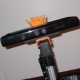ROS Resources: Documentation | Support | Discussion Forum | Index | Service Status | Q&A answers.ros.org

# Move base issue motor direction

Update: When move base sends a cmd_vel that is translated to say -1,-1 on the wheels the gazebo model moves backwards, but in rviz it moves forward. If I negate the values then the model moves forward but backwards in rviz. What would cause this?

Here are my setting also.

rrbot:
# Publish all joint states -----------------------------------
joint_state_controller:
type: joint_state_controller/JointStateController
publish_rate: 50

# Position Controllers ---------------------------------------
joint1_position_controller:
type: effort_controllers/JointVelocityController
joint: joint1
pid: {p: 30, i: 1, d: 1, i_clamp: 100}
joint2_position_controller:
type: effort_controllers/JointVelocityController
joint: joint2
pid: {p: 30, i: 1, d: 1, i_clamp: 100}

TrajectoryPlannerROS:
# for details see: <a href="http://www.ros.org/wiki/base_local_planner">http://www.ros.org/wiki/base_local_planner</a>
max_vel_x: .005
max_trans_vel: .05
min_vel_x: .05
min_trans_vel: .05
max_rotational_vel: .025   # 0.1 rad/sec = 5.7 degree/sec
min_in_place_rotational_vel: .025

acc_lim_th: .005
acc_lim_x:  .005
acc_lim_y:  0


More data on inversion:

[ INFO] [1381338035.462489594, 4.700000000]: pv1=[-0.913283] pv2=[-0.913356]
[ INFO] [1381338035.462557027, 4.700000000]: er1=[-0.086717] er2=[-0.086644]
[ INFO] [1381338035.462593604, 4.700000000]: sp1=[-1.000000] sp2=[-1.000000]

[ INFO] [1381338036.459313255, 4.800000000]: pv1=[-0.908281] pv2=[-0.912306]
[ INFO] [1381338036.459382353, 4.800000000]: er1=[1.408281] er2=[1.412306]
[ INFO] [1381338036.459414965, 4.800000000]: sp1=[0.500000] sp2=[0.500000]


Update: I have been seeing this now for a while. Move base will send a positive velocity on a wheel and in the very next sequence send a negative one or vice verse. Is there any configuration that will stop navigation from doing this. Not all motors can change direction like this. Would this be a new feature? Does anyone else see this behavior in move base?

I am running move base navigation and got the following which is causing a problem for the motor controls. I am not sure why this is happening could be initial odometry but in either case. I am using the gazebo ros velocity controller.

The navigation starts sending cmd velocities of -5 -5 then it switches to 1 1. Which cause the system to react violently. I would like a smooth transition so that the wheels do get thrown into reverse. notice the error continues to build even though the set point stays steady after the initial values.

The numbers I get are as follows from the joint state controller msg.

pv=process_value

er=error

sp=set_point

pv1=[-0.057762] pv2=[-0.045411]
er1=[0.057762] er2=[0.045411]
sp1=[0.000000] sp2=[0.000000]

pv1=[-0.339482] pv2=[-0.292756]
er1=[-0.160518] er2=[-0.207244]
sp1=[-0.500000] sp2=[-0.500000]

pv1=[-0.406273] pv2=[-0.389512]
er1=[-0.093727] er2=[-0.110488]
sp1=[-0.500000] sp2=[-0.500000]

pv1=[-0.111645] pv2=[-0.368285]
er1=[1.111645] er2=[1.368285]
sp1=[1.000000] sp2=[1.000000]

pv1=[-0.115296] pv2=[-0.294882]
er1=[1.115296] er2=[1.294882]
sp1=[1.000000] sp2=[1.000000]

pv1=[-0.209185] pv2=[-0.315181]
er1=[1.209185] er2=[1.315181]
sp1=[1.000000] sp2=[1.000000 ...
edit retag close merge delete

max_vel_x < min_vel_x.... i have no idea what that might do to the planner.

wow...didn't see that. Would be nice to have semantic checks. Thanks!

Sort by » oldest newest most votedYou should probably look into setting the acc_lim_x and acc_lim_th acceleration limits for the local planner. You should lower these enough to be within the limits of your robot.

more

This seem to stopped them from moving in opposite directions however I made other changes as well.